Related Articles

# Self -attention in NLP

• Last Updated : 05 Sep, 2020

Self-attention was proposed by researchers at Google Research and Google Brain. It was proposed due to challenges faced by encoder-decoder in dealing with long sequences. The authors also provide two variants of attention and transformer architecture. This transformer architecture generates the state-of-the-art results on WMT translation task.

Encoder-Decoder model:

Attention reader! Don’t stop learning now. Get hold of all the important Machine Learning Concepts with the Machine Learning Foundation Course at a student-friendly price and become industry ready.

The encoder-decoder model was presented in different papers. The difference between these two papers are on the basis of the relationship between input and output length

From a high-level, the model comprises two sub-models: an encoder and a decoder.

• Encoder: The encoder is responsible for stepping through the input time steps and encoding the entire sequence into a fixed-length vector called a context vector.
• Decoder: The decoder is responsible for stepping through the output time steps while reading from the context vector.

Attention mechanism :

Attention was proposed by authors of the Encoder-Decoder network as an extension of it. It is proposed to overcome the limitation of the Encoder-Decoder model encoding the input sequence to one fixed-length vector from which to decode each output time step. This issue is believed to be more of a problem when decoding long sequences.

Self-attention mechanism: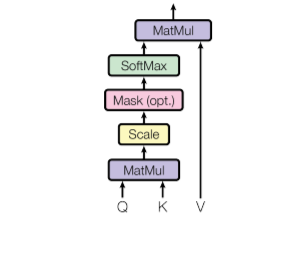Self-Attention

The attention mechanism allows output to focus attention on input while producing output while the self-attention model allows inputs to interact with each other (i.e calculate attention of all other inputs wrt one input.

• The first step is multiplying each of the encoder input vectors with three weights matrices (W(Q), W(K), W(V)) that we trained during the training process. This matrix multiplication will give us three vectors for each of the input vector: the key vector, the query vector, and the value vector.
• The second step in calculating self-attention is to multiply the Query vector of the current input with the key vectors from other inputs.
• In the third step, we will divide the score by square root of dimensions of the key vector (dk). In the paper the dimension of the key vector is 64, so that will be 8. The reason behind that is if the dot products become large, this causes some self-attention scores to be very small after we apply softmax function in the future.
• In the fourth step, we will apply the softmax function on all self-attention scores we calculated wrt the query word (here first word).
• In the fifth step, we multiply the value vector on the vector we calculated in the previous step.
• In the final step, we sum up the weighted value vectors that we got in the previous step, this will give us the self-attention output for the given word.

The above procedure is applied to all the input sequences. Mathematically, the self-attention matrix for input matrices (Q, K, V) is calculated as:where Q, K, V are the concatenation of query, key, and value vectors.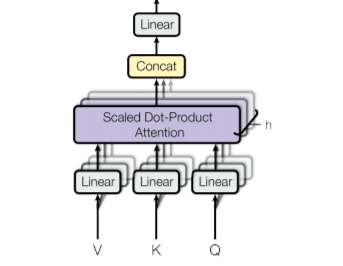In the attention paper, the authors proposed another type of attention mechanism called multi-headed attention. Below is the step-by-step process to calculate multi-headed self-attention:

• Take each word of input sentence and generate the embedding from it.
• In this mechanism, we created h (h = 8) different attention heads, each head has different weight matrices (W(Q), W(K), W(V)).
• In this step, we multiply the input matrix with each of the weight matrices (WQ, WK, WV) to produce the key, value, and query matrices for each attention head.
• Now, we apply the attention mechanism to these query, key, and value matrices, this gives us an output matrix from each attention head.
• In this step, we concatenate the output matrix obtained from each attention heads and dot product with the weight WO to generate the output of the multi-headed attention layer.

Mathematically multi-head attention can be represented by:Attention in Transformer architecture: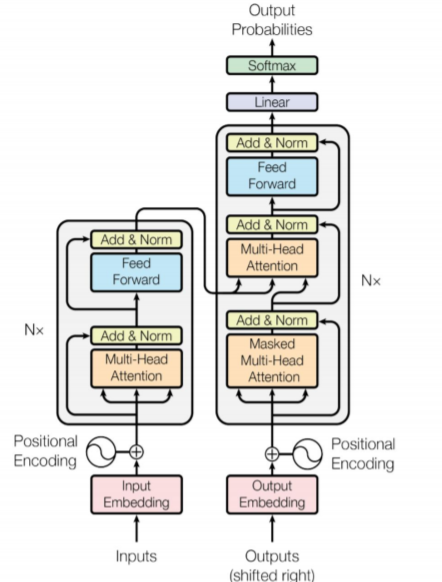The transformer architecture uses attention model uses multi-headed attention at three steps:

• The first is encoder-decoder attention layers, in this type of layer the queries come from the previous decoder layer while the keys and values come from the encoder output. This allows each position in the decoder to give attention to all the positions of the input sequence.
• The second type is the self-attention layer contained in the encoder, this layer receives key, value, and query input from the output of the previous encoder layer. Each position in the encoder can get attention score from every position in the previous encoder layer.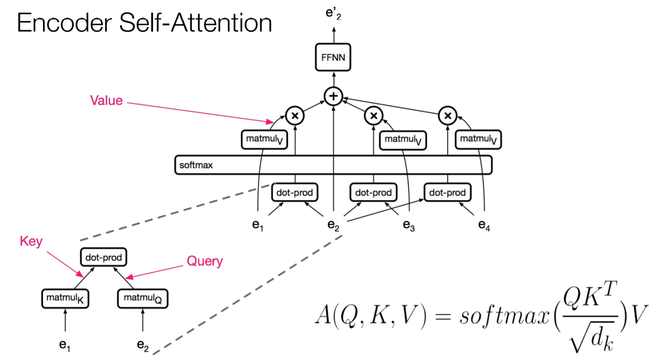Self-attention in Encoder

• The third type is the self-attention in the decoder, this is similar to self-attention in encoder where all queries, keys, and values come from the previous layer. The self-attention decoder allows each position to attend each position up to and including that position. The future values are masked with (-Inf). This is known as masked-self attention.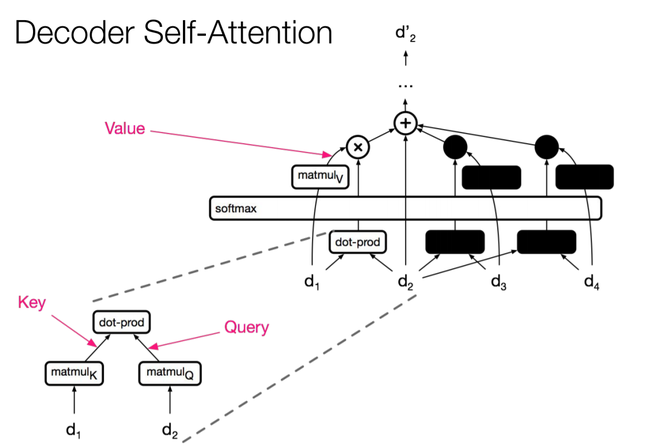Self-attention in Decoder

Complexity and Results:

The advantage of using self-attention layers in the NLP tasks that it is less computationally expensive to perform than other operations. Below is the table representing the complexity of different operations: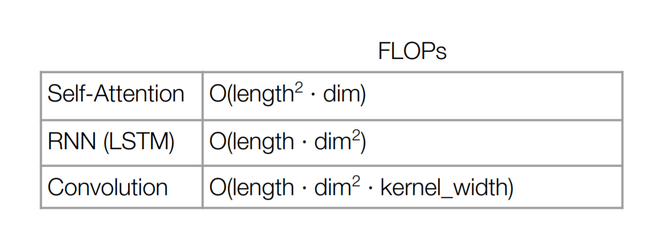Results:

• On the WMT 2014 English-to-German translation task, the transformer model achieves the BLUE score of 28.4 which is the new state-of-the-art in this task. The model is trained for 3.5 days on 8 NVIDIA P100 GPUs. This is at least three times faster than previous state-of-the-art models.
• On the WMT 2014 English-to-French translation task, the model achieved a BLUE score 41.8, which is also the new state-of-the-art result in this task but with a fraction of training cost.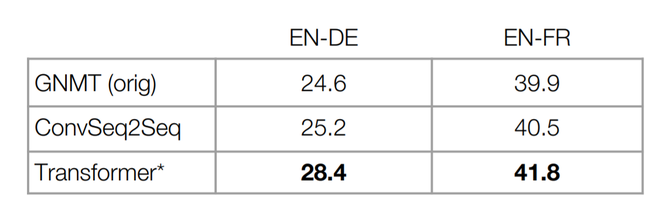References:

My Personal Notes arrow_drop_up